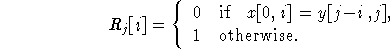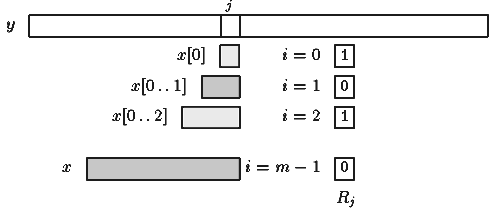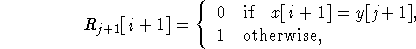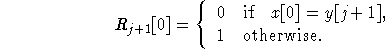Name
• String
• Substring
• Search
Edit
he Shift Or algorithm uses bitwise techniques. Let R be a bit array of size m. Vector Rj is the value of the array R after text character y[j] has been processed (see figure 5.1). It contains informations about all matches of prefixes of x that end at position j in the text for 0 < i  m-1:Figure 5.1: Meaning of vector Rj.

The vector Rj+1 can be computed after Rj as follows. For each Rj[i]=0:andIf Rj+1[m-1]=0 then a complete match can be reported.

The transition from Rj to Rj+1 can be computed very fast as follows: For each c in , let Sc be a bit array of size m such that: for 0  i < m-1, Sc[i]=0 iff x[i]=c.

The array Sc denotes the positions of the character c in the pattern x. Each Sc can be preprocessed before the search. And the computation of Rj+1 reduces to two operations, shift and or: Rj+1=SHIFT(Rj) OR Sy[j+1]

Assuming that the pattern length is no longer than the memory-word size of the machine, the space and time complexity of the preprocessing phase is O(m+q).

The time complexity of the searching phase is O(n), thus independent from the alphabet size and the pattern length.

Main features:

• uses bitwise techniques;
• efficient if the pattern length is no longer than the memory-word size of the machine;
• preprocessing phase in O(m + ) time and space complexity;
• searching phase in O(n) time complexity (independent from the alphabet size and the pattern length);
• adapts easily to approximate string matching.
C